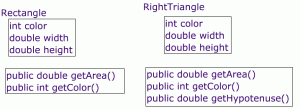# Question: What limitations seem to exist with our use of classes?

I. Implement all Methods of the Triangle and Rectangle methods belowClass: RectangleRectangle

Constructor

• public Rectangle ( int  _col,  double  _width,  double _height)

instance Variables

• private int color;
• private double width;
• private double height ;

Accessor Methods

• public double getArea(); //returns the area of the rectangle
• public int getColor()

### Class: RightTriangleRightTriangle

Constructor

• public RightTriangle( int _col,  double  _width,  double _height)

instance Variables

• private int color;
• private double width;
• private double height;

Accessor Methods

• public double getArea(); //returns the area of the triangle
• public int getColor()
• public double getHypotenuse() ;

### Class: InteractiveMath

instance Variables

• ArrayList<Rectangle> rectangles;
• ArrayList<RightTriangle> triangles;

Accesor Methods

• public double computeNetArea(){ //@ returns the area of the rectangles and triangles

Next  : Super Classes are great 2

Then  : constructors and inheritance.

after that : What is “Inherited” by Subclasses?

interactiveMath_arrays